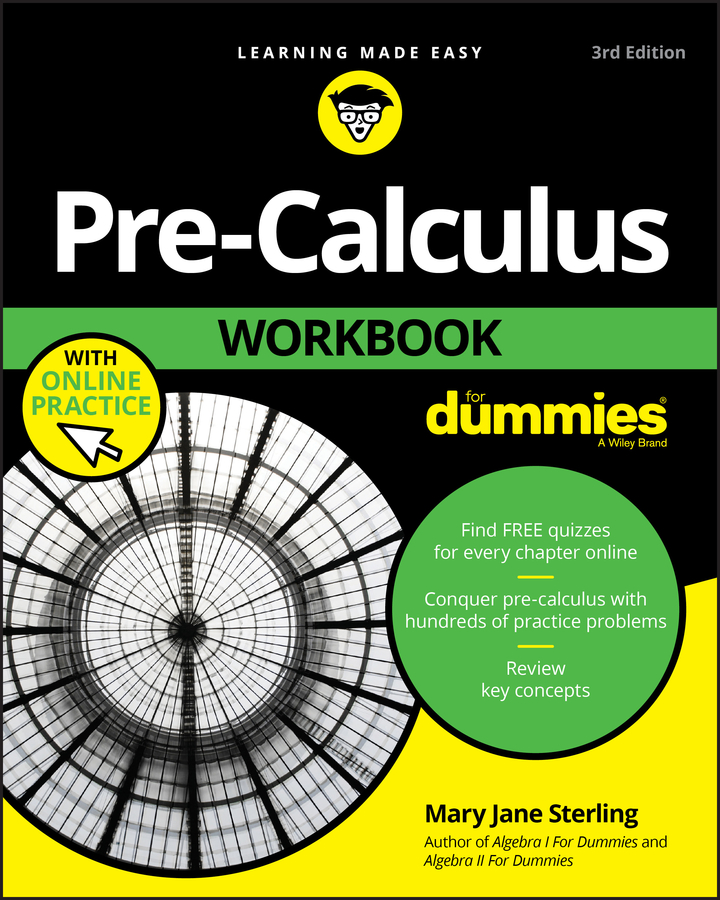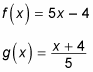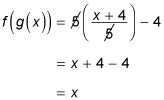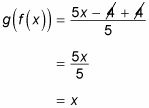##### Pre-Calculus Workbook For DummiesAt times, your textbook or teacher may ask you to verify that two given functions are actually inverses of each other. To do this, you need to show that both f(g(x)) and g(f(x)) = x.

When you’re asked to find an inverse of a function, you should verify on your own that the inverse you obtained was correct, time permitting.

For example, show that the following functions are inverses of each other:1. Show that f(g(x)) = x.

This step is a matter of plugging in all the components:2. Show that g(f(x)) = x.

Again, plug in the numbers and start crossing out: